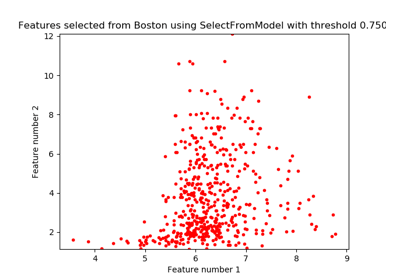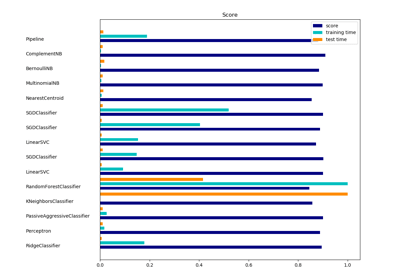/scikit-learn

# sklearn.feature_selection.SelectFromModel

`class sklearn.feature_selection.SelectFromModel(estimator, threshold=None, prefit=False, norm_order=1, max_features=None)` [source]

Meta-transformer for selecting features based on importance weights.

New in version 0.17.

Parameters: `estimator : object` The base estimator from which the transformer is built. This can be both a fitted (if `prefit` is set to True) or a non-fitted estimator. The estimator must have either a `feature_importances_` or `coef_` attribute after fitting. `threshold : string, float, optional default None` The threshold value to use for feature selection. Features whose importance is greater or equal are kept while the others are discarded. If “median” (resp. “mean”), then the `threshold` value is the median (resp. the mean) of the feature importances. A scaling factor (e.g., “1.25*mean”) may also be used. If None and if the estimator has a parameter penalty set to l1, either explicitly or implicitly (e.g, Lasso), the threshold used is 1e-5. Otherwise, “mean” is used by default. `prefit : bool, default False` Whether a prefit model is expected to be passed into the constructor directly or not. If True, `transform` must be called directly and SelectFromModel cannot be used with `cross_val_score`, `GridSearchCV` and similar utilities that clone the estimator. Otherwise train the model using `fit` and then `transform` to do feature selection. `norm_order : non-zero int, inf, -inf, default 1` Order of the norm used to filter the vectors of coefficients below `threshold` in the case where the `coef_` attribute of the estimator is of dimension 2. `max_features : int or None, optional` The maximum number of features selected scoring above `threshold`. To disable `threshold` and only select based on `max_features`, set `threshold=-np.inf`. New in version 0.20. `estimator_ : an estimator` The base estimator from which the transformer is built. This is stored only when a non-fitted estimator is passed to the `SelectFromModel`, i.e when prefit is False. `threshold_ : float` The threshold value used for feature selection.

#### Methods

 `fit`(X[, y]) Fit the SelectFromModel meta-transformer. `fit_transform`(X[, y]) Fit to data, then transform it. `get_params`([deep]) Get parameters for this estimator. `get_support`([indices]) Get a mask, or integer index, of the features selected `inverse_transform`(X) Reverse the transformation operation `partial_fit`(X[, y]) Fit the SelectFromModel meta-transformer only once. `set_params`(**params) Set the parameters of this estimator. `transform`(X) Reduce X to the selected features.
`__init__(estimator, threshold=None, prefit=False, norm_order=1, max_features=None)` [source]
`fit(X, y=None, **fit_params)` [source]

Fit the SelectFromModel meta-transformer.

Parameters: `X : array-like of shape (n_samples, n_features)` The training input samples. `y : array-like, shape (n_samples,)` The target values (integers that correspond to classes in classification, real numbers in regression). `**fit_params : Other estimator specific parameters` `self : object`
`fit_transform(X, y=None, **fit_params)` [source]

Fit to data, then transform it.

Fits transformer to X and y with optional parameters fit_params and returns a transformed version of X.

Parameters: `X : numpy array of shape [n_samples, n_features]` Training set. `y : numpy array of shape [n_samples]` Target values. `X_new : numpy array of shape [n_samples, n_features_new]` Transformed array.
`get_params(deep=True)` [source]

Get parameters for this estimator.

Parameters: `deep : boolean, optional` If True, will return the parameters for this estimator and contained subobjects that are estimators. `params : mapping of string to any` Parameter names mapped to their values.
`get_support(indices=False)` [source]

Get a mask, or integer index, of the features selected

Parameters: `indices : boolean (default False)` If True, the return value will be an array of integers, rather than a boolean mask. `support : array` An index that selects the retained features from a feature vector. If `indices` is False, this is a boolean array of shape [# input features], in which an element is True iff its corresponding feature is selected for retention. If `indices` is True, this is an integer array of shape [# output features] whose values are indices into the input feature vector.
`inverse_transform(X)` [source]

Reverse the transformation operation

Parameters: `X : array of shape [n_samples, n_selected_features]` The input samples. `X_r : array of shape [n_samples, n_original_features]` `X` with columns of zeros inserted where features would have been removed by `transform`.
`partial_fit(X, y=None, **fit_params)` [source]

Fit the SelectFromModel meta-transformer only once.

Parameters: `X : array-like of shape (n_samples, n_features)` The training input samples. `y : array-like, shape (n_samples,)` The target values (integers that correspond to classes in classification, real numbers in regression). `**fit_params : Other estimator specific parameters` `self : object`
`set_params(**params)` [source]

Set the parameters of this estimator.

The method works on simple estimators as well as on nested objects (such as pipelines). The latter have parameters of the form `<component>__<parameter>` so that it’s possible to update each component of a nested object.

Returns: self
`transform(X)` [source]

Reduce X to the selected features.

Parameters: `X : array of shape [n_samples, n_features]` The input samples. `X_r : array of shape [n_samples, n_selected_features]` The input samples with only the selected features.

## Examples using `sklearn.feature_selection.SelectFromModel`Feature selection using SelectFromModel and LassoCVClassification of text documents using sparse features